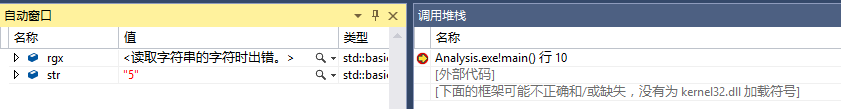C++使用正则表达式出现错误``````#include <string>
#include <iostream>
#include <regex>
#include <stdio.h>

int main()
{
std::string str = "20110604-092737<INFO :0xb7f2a6d0>: Player logout: 忘了爱过谁";
std::regex rgx("^[\\d\\-\\d\\<\\w\\s{0,1}\\:\\w>]");
std::smatch result;
if (std::regex_search(str, result, rgx))
{
std::cout << result.str();
}

}

``````

4个回答

``````    std::regex rgx("^\\d*-\\d*<\\w*\\s{0,1}:\\w*>");

``````ysuwood 回复caozhy: 楼主意图还是很明显的，按他写的格式能看出来。ysuwood 楼主意图还是很明显的，按他写的格式能看出来。`````` \\d{8}\\-\\d{6}\\<\\\\INFO\\s\\:0x[0-9a-fA-F]{8}\\>
``````
``````    std::regex rgx("^[\\d]*[-][\\d]*[<][\\w]*[\\s{0,1}][:][\\w]*[>]");

``````

``````    std::regex rgx("\\d*-\\d*<\\w* :\\w*>");

``````